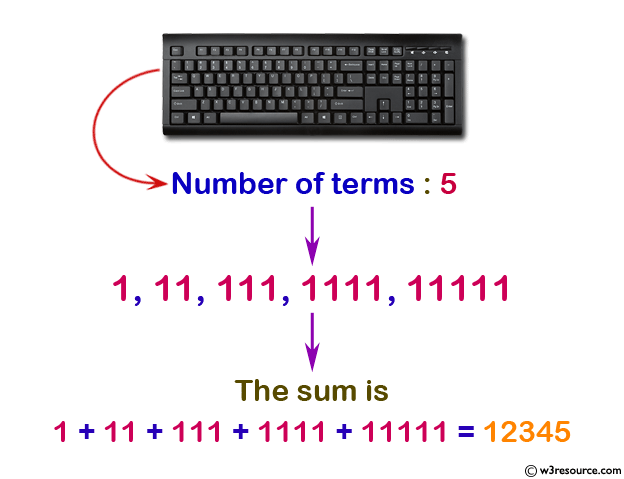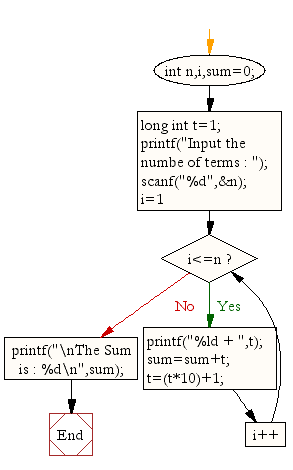﻿ C : Calculate the sum of the series 1 +11 + 1111 + ... N# C Exercises: Calculate the sum of the series 1 +11 + 111 + 1111 + .. n terms

## C For Loop: Exercise-26 with Solution

Write a program in C to find the sum of the series 1 +11 + 111 + 1111 + .. n terms.

Pictorial Presentation:Sample Solution:

C Code:

``````#include <stdio.h>

void main()
{
int n,i;
long sum=0;
long int t=1;
printf("Input the number of terms : ");
scanf("%d",&n);
for(i=1;i<=n;i++)
{
printf("%ld  ",t);
if (i<n)
{
printf("+ ");

}
sum=sum+t;
t=(t*10)+1;
}
printf("\nThe Sum is : %ld\n",sum);
}
```
```

Sample Output:

```Input the number of terms : 5
1 + 11 + 111 + 1111 + 11111
The Sum is : 12345
```

Flowchart:C Programming Code Editor:

Improve this sample solution and post your code through Disqus.

What is the difficulty level of this exercise?

Test your Programming skills with w3resource's quiz.

﻿

## C Programming: Tips of the Day

How to initialize array to 0 in C?

Global variables and static variables are automatically initialized to zero. If you have simply-

`char ZEROARRAY;`

at global scope it will be all zeros at runtime. But actually there is a shorthand syntax if you had a local array. If an array is partially initialized, elements that are not initialized receive the value 0 of the appropriate type. You could write:

`char ZEROARRAY = {0};`

The compiler would fill the unwritten entries with zeros. Alternatively you could use memset to initialize the array at program startup:

Ref : https://bit.ly/3cvqplP